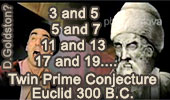# Euclid's Elements - Table of ContentEuclid's Elements is a mathematical and geometric treatise consisting of 13 books written by the Greek mathematician Euclid in Alexandria circa 300 BC. It comprises a collection of definitions, postulates (axioms), propositions (theorems and constructions), and proofs.

Euclid's Elements Book I, 23 Definitions.
One-page visual illustration.

Euclid's Elements Book I, Proposition 6: Converse of I.5

Euclid's Elements Book I, Proposition 7
Given two straight lines constructed on a straight line (from its extremities) and meeting in a point,...

The significance of the Pythagorean theorem  by Jacob Bronowski.
Pythagorean Theorem, 47th Proposition of Euclid's Book I.

Euclid's Elements Book V Word Cloud.
A treatise on proportions of magnitudes. Proposition 25 has as a special case the inequality of arithmetic and geometric means.

Euclid's Elements Book IV Word Cloud.
Constructs the incircle and circumcircle of a triangle, and constructs regular polygons with 4, 5, 6, and 15 sides

Euclid's Elements, Book X, Lemma for Proposition 33 One page visual illustration.

Euclid's Elements, Book XIII, Proposition 10 One page visual illustration.

MindMap of Euclid's Elements. A mindmap is an excellent learning tool for visual communication, organization, content sequencing, and navigation on Internet.

The School of Athens Euclid and Pythagoras together!
VideoTwin Prime Conjecture. is a famous unsolved problem in number theory discovered by Euclid.